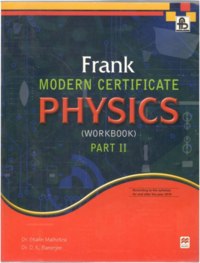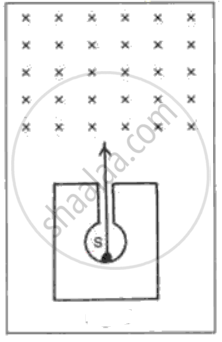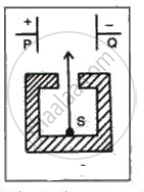# Frank solutions for ICSE Class 10 Physics Part 2 chapter 6 - Modern Physics [Latest edition]

#### Chapters## Chapter 6: Modern Physics

Exercise 6.1Exercise
Exercise 6.1 [Page 261]

### Frank solutions for ICSE Class 10 Physics Part 2 Chapter 6 Modern Physics Exercise 6.1 [Page 261]

Exercise 6.1 | Q 1 | Page 261
Name the three elementary particles present in an atom. Locate their positions.
Exercise 6.1 | Q 2 | Page 261
Define nucleus.
Exercise 6.1 | Q 3.1 | Page 261

What do you mean by Atomic number

Exercise 6.1 | Q 3.2 | Page 261

What do you mean by Mass number.

Exercise 6.1 | Q 3.3 | Page 261

What do you mean by Atomic mass

Exercise 6.1 | Q 4.1 | Page 261

State the penetrating range of α, β and γ radiations.

Exercise 6.1 | Q 4.2 | Page 261

Give the relative ionizing power of α, β and γ radiations.

Exercise 6.1 | Q 5 | Page 261

Which of the radiations α, β and γ is similar to a beam of electrons?

Exercise 6.1 | Q 6 | Page 261
What are isotopes?
Exercise 6.1 | Q 7 | Page 261
Do isotopes have same chemical or physical properties?
Exercise 6.1 | Q 8 | Page 261
What are isobars?
Exercise 6.1 | Q 9 | Page 261

State two similarities and two dissimilarities between the γ-rays and X-rays.

Exercise 6.1 | Q 10 | Page 261

What happens to the position of an element in the periodic table when it

Exercise 6.1 | Q 11 | Page 261

What happens to the atomic number of element when (i) An α -particle, (ii) A β -particle 1 and (iii)  γ-radiation is emitted?

Exercise 6.1 | Q 12 | Page 261

What happens to the mass number of an element when (i) An  α -particle, (ii) A β -particle, and (iii)  γ -radiation is emitted?

Exercise 6.1 | Q 13 | Page 261

(i) Name the radiations which are charged.
(ii) Name the radiations which are most penetrating.
(iii) Name the radiations which travel with the speed of light.
(iv) Name the radiations which have the largest mass.

Exercise 6.1 | Q 14 | Page 261
'Radioactivity is a nuclear phenomenon'. Comment on this statement.
Exercise 6.1 | Q 15 | Page 261

What kind of change takes place in a nucleus when a β -particle is emitted?

Exercise 6.1 | Q 16 | Page 261

In following fig.  shows a mixed source S of alpha and beta particles in a thick lead container. The particles pass through a magnetic field in a direction perpendicular to the plane of paper (inwards as shown by x). State and show in the diagram how the particles get affected.Exercise 6.1 [Page 262]

### Frank solutions for ICSE Class 10 Physics Part 2 Chapter 6 Modern Physics Exercise 6.1 [Page 262]

Exercise 6.1 | Q 1 | Page 262

In the following  fig. shows a radioactive source S in a thick lead container. The radiations pass through an electric field between the plates P and Q. Complete the diagram to show the paths of α , β and γ radiations.Why is the source S placed in a thick lead container?

Exercise 6.1 | Q 2 | Page 262

A nucleus of an element X which has the symbol ""_84^202 X emits an alpha particle and then a beta particle. The final nucleus is ""_"b"^"a" Y Find a and b.

Exercise 6.1 | Q 3 | Page 262
The mass number (A) of an element is not changed when it emits ______________.
Exercise 6.1 | Q 4 | Page 262

The atomic number of a radioactive element is not changed when it emits ............

Exercise 6.1 | Q 5 | Page 262

A radioactive nucleus ""_"Z"^"A" X first emits a beta particle and then an alpha particle to give the resulting nucleus ""_"Q"^"P" Y  What will be the values of P and Q in terms of A and Z?

Exercise 6.1 | Q 6 | Page 262
Exercise 6.1 | Q 7 | Page 262
State three safety precautions that you would take while handling the radioactive substances.
Exercise 6.1 | Q 8 | Page 262
Why should a radioactive substance not be touched by hand?
Exercise [Page 266]

### Frank solutions for ICSE Class 10 Physics Part 2 Chapter 6 Modern Physics Exercise [Page 266]

Exercise | Q 1.1 | Page 266

Make the correct choices in the following:
(a) In one atom of copper (mass number 63, atomic number 29) there are

• 63 protons and 29 neutrons.

• 63 protons and 29 electrons.

• 29 protons and 29 neutrons.

• 29 protons and 29 electrons.

• 29 protons and 63 neutrons.

Exercise | Q 1.2 | Page 266

A radioactive nuclide ""_86^226 Ra decays by emission of two alpha particles, one beta particle and gamma rays. Which of the following is the resulting nuclide X?

• 223 x 83

• 218 x 83

• 218 x 84

• 217 x 85

• 218 x 85

Exercise | Q 1.3 | Page 266

The element X has an atomic mass number of 238 and an atomic number
of 92. It emits an alpha particle forming an element y. Y can be represented
by

• 234 x 90

• 236 x 90

• 235 x 91

• 238 x 92

• 238 x 934

Exercise | Q 1.4 | Page 266

An element P has an atomic mass of 239 and an atomic number of 92. It
emits a beta particle forming an element Q. Q can be represented by

• 239 x 91

• 239 x 92

• 239 x 93

• 238 x 92

• 235 x 90

Exercise | Q 1.5 | Page 266

Which one of the following statements about alpha and beta particles is
correct?

• They carry equal amounts of charge.

• Each alpha particle has four times the mass of a beta particle.

• Only alpha particles can cause ionization

• They can both be deflected by magnetic fields when they are in
motion.

• Alpha particles have a larger range in air than beta particles.

Exercise [Page 267]

### Frank solutions for ICSE Class 10 Physics Part 2 Chapter 6 Modern Physics Exercise [Page 267]

Exercise | Q 1 | Page 267

(a) Which has a positive charge?
(b) Which is most penetrating?
(c) Which has no electric charge?

Exercise | Q 2.1 | Page 267

Which of the following statements are correct?
Electrons have

• No mass,

• A mass less than that of a proton,

•  A mass equal to that of a helium atom,

• Mass greater than that of a neutron.

Exercise | Q 2.2 | Page 267

Which of the following statements are correct?

Neutrons have

•  A double positive charge,

• A single positive charge,

•  A negative charge,

• No electric charge.

Exercise | Q 3 | Page 267

Draw a diagram of a simple atom showing the nucleus and electrons. In this atom:
(a) What type of charge will there be on the nucleus?
(b) What is the value of this charge?

Exercise | Q 4 | Page 267
What are the names of the three types of rays given off by a radioactive substance? Define the properties you know they possess. Why are the largest atoms radioactive?
Exercise | Q 5 | Page 267

One isotopes of uranium has a mass number of 235 and atomic number 92.
(a) What is the number of electrons in a neutral atom of this isotope?

(b) How many protons are there in the nucleus of this isotope?

(c) For another isotope of Uranium state which one of the numbers (238 or 92) changes?

(d) What is the number of protons in 238U?

Exercise | Q 6 | Page 267

Radioactive substances were found to give off three types of rays. Name them. How do they
(a) React to the magnetic field?
(b) React to the electric field?
(c) Act when different thickness of lead sheets is placed in their path?

Exercise | Q 7 | Page 267
Chlorine has an atomic number 17 in the form of isotope, one of atomic weight 35 and the other atomic weight 37. What do you understand by an isotope? Name two other elements which have isotopes.
Exercise | Q 8.1 | Page 267

What is the meaning of mass number?

Exercise | Q 8.2 | Page 267

What do you mean by atomic number?

Exercise | Q 8.3 | Page 267

In what way are these numbers related to the isotopes of an element?

Exercise | Q 8.4 | Page 267

Explain the use of radioactive in the field of medicine, agriculture and industry.

Exercise | Q 9 | Page 267

Distinguish between α, β and γ rays.

Exercise | Q 10 | Page 267

What do you understand by radioactive decay? An isotope of Uranium ""_92^238 "U"  is radioactive and changes into Thorium 92 by the emission of an alpha particle. Write a symbolic equation for this decay process.

Exercise | Q 11 | Page 267

The element  ""_11^24 "Na"  changes to magnesium by the emission of a beta particle. Write down the symbolic equation for the beta emission.

Exercise | Q 12 | Page 267

What are the uses of radioactive isotopes in following?
(a) Medical field,
(b) Agriculture,
(c) Industries?

Exercise | Q 13 | Page 267
What do you mean by background radiations? Name its sources. Is it possible for us to keep ourselves away from it?
Exercise | Q 14 | Page 267
State some of the safety precautions that we have to taken when we are exposed to some of the radiations or when we are handling some of the radioactive substances.

## Chapter 6: Modern Physics

Exercise 6.1Exercise## Frank solutions for ICSE Class 10 Physics Part 2 chapter 6 - Modern Physics

Frank solutions for ICSE Class 10 Physics Part 2 chapter 6 (Modern Physics) include all questions with solution and detail explanation. This will clear students doubts about any question and improve application skills while preparing for board exams. The detailed, step-by-step solutions will help you understand the concepts better and clear your confusions, if any. Shaalaa.com has the CISCE ICSE Class 10 Physics Part 2 solutions in a manner that help students grasp basic concepts better and faster.

Further, we at Shaalaa.com provide such solutions so that students can prepare for written exams. Frank textbook solutions can be a core help for self-study and acts as a perfect self-help guidance for students.

Concepts covered in ICSE Class 10 Physics Part 2 chapter 6 Modern Physics are Nuclear Energy, Radiation, Safety Precautions While Using Nuclear Energy, Nuclear Fission, Nuclear Fusion, Distinction Between the Radioactive Decay and Nuclear Fission, Distinction Between the Nuclear Fission and Nuclear Fusion, Atoms: Building Blocks of Matter, Structure of an Atom, Discovery of Charged Particles in Matter, Nucleus, Atomic Mass, Atomic Number (Z), Mass Number (A), and Number of Neutrons (n), Isotopes, Isobars, Isotones, Radioactivity, Radioactivity as Emission of Alpha, Beta, and Gamma Radiations, Properties of Alpha Particles, Properties of Beta Particles, Properties of Gamma Radiations, Changes Within the Nucleus in Alpha, Beta and Gamma Emission, Alpha Decay (Alpha Emission), Beta Decay (Beta Emission), Gamma Decay (Gamma Emission), Uses of Radioactivity.

Using Frank Class 10 solutions Modern Physics exercise by students are an easy way to prepare for the exams, as they involve solutions arranged chapter-wise also page wise. The questions involved in Frank Solutions are important questions that can be asked in the final exam. Maximum students of CISCE Class 10 prefer Frank Textbook Solutions to score more in exam.

Get the free view of chapter 6 Modern Physics Class 10 extra questions for ICSE Class 10 Physics Part 2 and can use Shaalaa.com to keep it handy for your exam preparation